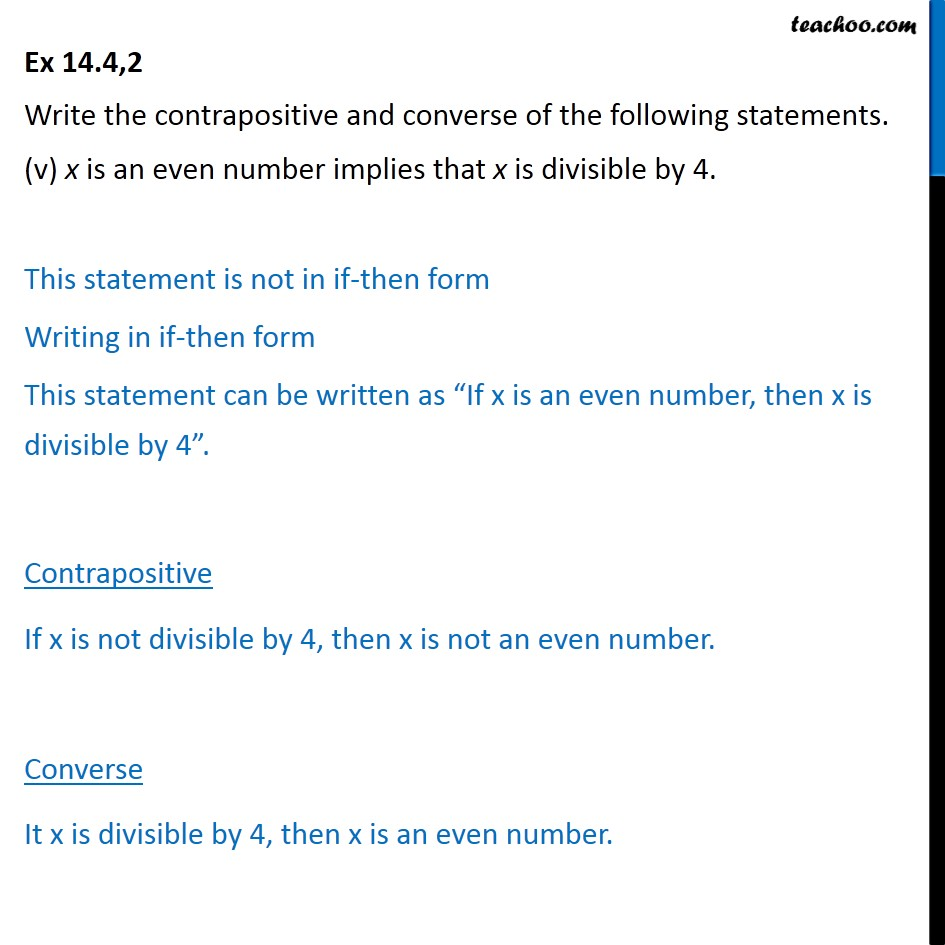If then Statements

Mathematical Reasoning
Serial order wiseLearn in your speed, with individual attention - Teachoo Maths 1-on-1 Class

### Transcript

Question2 Write the contrapositive and converse of the following statements. (v) x is an even number implies that x is divisible by 4. This statement is not in if-then form Writing in if-then form This statement can be written as “If x is an even number, then x is divisible by 4”. Contrapositive If x is not divisible by 4, then x is not an even number. Converse It x is divisible by 4, then x is an even number.